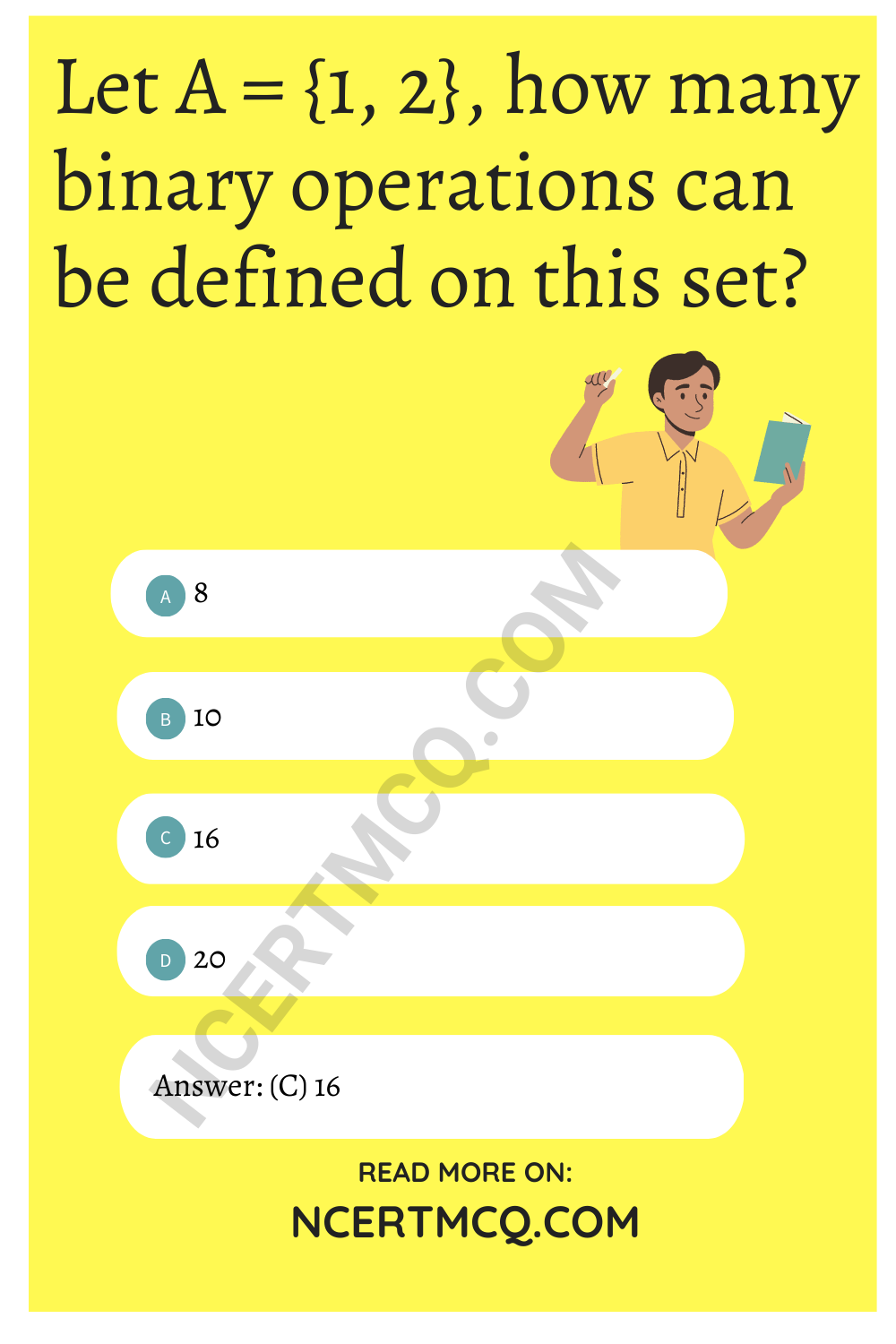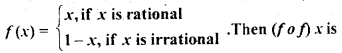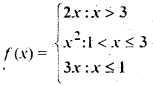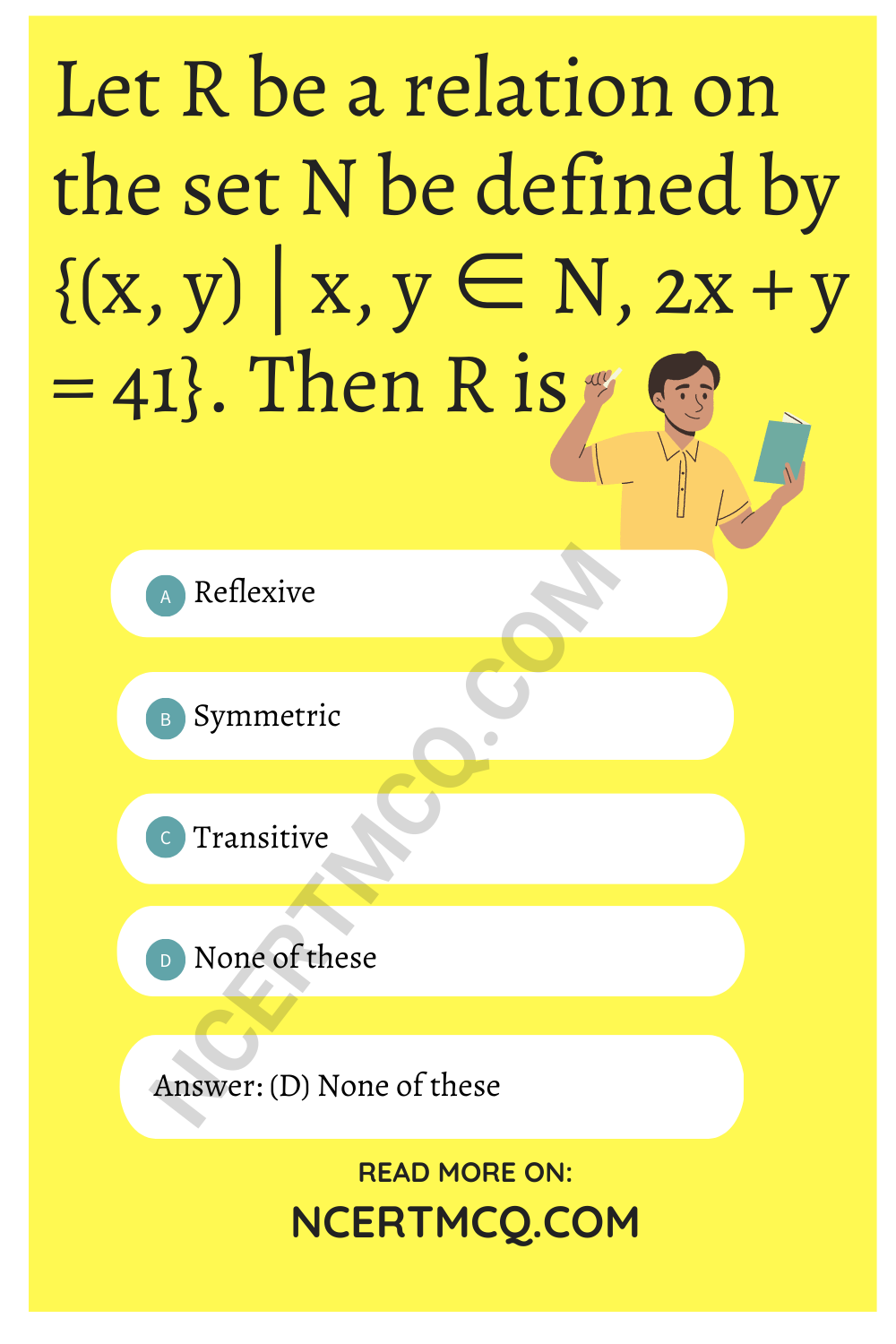Check the below NCERT MCQ Questions for Class 12 Maths Chapter 1 Relations and Functions with Answers Pdf free download. MCQ Questions for Class 12 Maths with Answers were prepared based on the latest exam pattern. We have provided Relations and Functions Class 12 Maths MCQs Questions with Answers to help students understand the concept very well.

## Relations and Functions Class 12 MCQs Questions with Answers

Relation And Function Class 12 MCQ Question 1.
If f(x1) = f (x2) ⇒ x1 = x2 ∀ x1 x2 ∈ A then the function f: A → B is
(a) one-one
(b) one-one onto
(c) onto
(d) many one

Relations And Functions Class 12 MCQ Question 2.
What type of a relation is R = {(1, 3), (4, 2), (2, 4), (2, 3), (3, 1)} on the set A – {1, 2, 3, 4}
(a) Reflexive
(b) Transitive
(c) Symmetric
(d) None of these

MCQ On Relation And Function Class 12 Question 3.
If F : R → R such that f(x) = 5x + 4 then which of the following is equal to f-1(x).
(a) $$\frac{x-5}{4}$$
(b) $$\frac{x-y}{5}$$
(c) $$\frac{x-4}{5}$$
(d) $$\frac{x}{4}$$ -5

Answer: (c) $$\frac{x-4}{5}$$

MCQ Of Relation And Function Class 12 Question 4.
If an operation is defined by a* b = a² + b², then (1 * 2) * 6 is
(a) 12
(b) 28
(c) 61
(d) None of these

Relation And Function MCQ Question 5.
Consider the binary operation * on a defined by x * y = 1 + 12x + xy, ∀ x, y ∈ Q, then 2 * 3 equals
(a) 31
(b) 40
(c) 43
(d) None of these

Class 12 Maths Chapter 1 MCQ Questions Question 6.
The range of the function f(x) = $$\sqrt{(x-1)(3-x)}$$ is
(a) [1, 3]
(b) [0, 1]
(c) [-2, 2]
(d) None of these

Relation And Function Class 12 MCQ Questions Question 7.
If f: R → R defined by f(x) = 2x + 3 then f-1(x) =
(a) 2x – 3
(b) $$\frac{x-3}{2}$$
(c) $$\frac{x+3}{2}$$
(d) None of these

Answer: (b) $$\frac{x-3}{2}$$

Class 12 Maths Chapter 1 MCQ Question 8.
The function f(x) = log (x² + $$\sqrt{x^2+1}$$ ) is
(a) even function
(b) odd function
(c) Both
(d) None of these

Relation And Function MCQ Class 12 Question 9.
Let E = {1, 2, 3, 4} and F = {1, 2} Then, the number of onto functions from E to F is
(a) 14
(b) 16
(c) 12
(d) 8

MCQ Of Maths Class 12 Chapter 1 Question 10.
If A, B and C are three sets such that A ∩ B = A ∩ C and A ∪ B = A ∪ C. then
(a) A = B
(b) A = C
(c) B = C
(d) A ∩ B = d

Relations And Functions MCQ Question 11.
Let A = {1, 2}, how many binary operations can be defined on this set?
(a) 8
(b) 10
(c) 16
(d) 20MCQ Relation And Function Class 12 Question 12.
Let A = {1, 2, 3, 4,…. n} How many bijective function f : A → B can be defined?
(a) $$\frac{1}{2}$$n(d) n

Class 12 Maths Ch 1 MCQ Questions Question 13.
If A = (1, 2, 3}, B = {6, 7, 8} is a function such that f(x) = x + 5 then what type of a function is f?
(a) Many-one onto
(b) Constant function
(c) one-one onto
(d) into

Relation Function Class 12 MCQ Question 14.
Let function R → R is defined as f(x) = 2x³ – 1, then ‘f’ is
(a) 2x³ + 1
(b) (2x)³ + 1
(c) (1 – 2x)³
(d) ($$\frac{1+x}{2}$$)1/3

Answer: (d) ($$\frac{1+x}{2}$$)1/3

Relation And Function Class 12 MCQ Term 1 Question 15.
Let the functioin ‘f’ be defined by f (x) = 5x² + 2 ∀ x ∈ R, then ‘f’ is
(a) onto function
(b) one-one, onto function
(c) one-one, into function
(d) many-one into function

MCQs On Relations And Functions Class 12 Question 16.
A relation R in human being defined as, R = {{a, b) : a, b ∈ human beings : a loves A} is-
(a) reflexive
(b) symmetric and transitive
(c) equivalence
(d) None of these

Class 12 Relation And Function MCQ Question 17.
If f(x) + 2f (1 – x) = x² + 2 ∀ x ∈ R, then f(x) =
(a) x² – 2
(b) 1
(c) $$\frac{1}{3}$$ (x – 2)²
(d) None of these

Answer: (c) $$\frac{1}{3}$$ (x – 2)²

MCQ Questions On Relations And Functions Class 12 Question 18.
The period of sin² θ is
(a) π²
(b) π
(c) 2π
(d) $$\frac{π}{2}$$

Class 12 Maths MCQ Chapter 1 Question 19.
The domain of sin-1 (log (x/3)] is. .
(a) [1, 9]
(b) [-1, 9]
(c) [-9, 1]
(d) [-9, -1]

Ch 1 Maths Class 12 MCQ Question 20.
f(x) = $$\frac{log_2(x+3)}{x^2+3x+2}$$ is the domain of
(a) R – {-1, -2}
(b) (- 2, ∞) .
(c) R- {- 1,-2, -3}
(d) (-3, + ∞) – {-1, -2}

Answer: (d) (-3, + ∞) – {-1, -2}

Class 12 Relations And Functions MCQ Question 21.
If the function f(x) = x³ + ex/2 and g (x) = fn(x), then the value of g'(1) is
(a) 1
(b) 2
(c) 3
(d) 4

Chapter 1 Maths Class 12 MCQ Question 22.
What type of relation is ‘less than’ in the set of real numbers?
(a) only symmetric
(b) only transitive
(c) only reflexive
(d) equivalence

Relations And Functions Class 12 MCQs Question 23.
If A = [1, 2, 3}, B = {5, 6, 7} and f: A → B is a function such that f(x) = x + 4 then what type of function is f?
(a) into
(b) one-one onto
(c) many-onto
(d) constant function

Relation And Function Class 12th MCQ Question 24.
f: A → B will be an into function if
(a) range (f) ⊂ B
(b) f(a) = B
(c) B ⊂ f(a)
(d) f(b) ⊂ A

Answer: (a) range (f) ⊂ B

Question 25.
If f : R → R such that f(x) = 3x then what type of a function is f?
(a) one-one onto
(b) many one onto
(c) one-one into
(d) many-one into

Question 26.
If f: R → R such that f(x) = 3x – 4 then which of the following is f-1(x)?
(a) $$\frac{1}{3}$$ (x + 4)
(b) $$\frac{1}{3}$$ (x – 4)
(c) 3x – 4
(d) undefined

Answer: (a) $$\frac{1}{3}$$ (x + 4)

Question 27.
A = {1, 2, 3} which of the following function f: A → A does not have an inverse function
(a) {(1, 1), (2, 2), (3, 3)}
(b) {(1, 2), (2, 1), (3, 1)}
(c) {(1, 3), (3, 2), (2, 1)}
(d) {(1, 2), (2, 3), (3, 1)

Answer: (b) {(1, 2), (2, 1), (3, 1)}

Question 28.
Let T be the set of all triangles in the Euclidean plane, and let a relation R on T be defined as aRb if a congruent to b ∀ a, b ∈ T. Then R is
(a) reflexive but-not transitive
(b) transitive but not symmetric
(c) equivalence
(d) None of these

Question 29.
Consider the non-empty set consisting of children is a family and a relation R defined as aRb If a is brother of b. Then R is
(a) symmetric but not transitive
(b) transitive but not symmetric
(c) neither symmetric nor transitive
(d) both symmetric and transitive

Answer: (b) transitive but not symmetric

Question 30.
The maximum number of equivalence relations on the set A = {1, 2, 3} are
(a) 1
(b) 2
(c) 3
(d) 5

Question 31.
If a relation R on the set {1, 2, 3} be defined by R = {(1, 2)}, then R is
(a) reflexive
(b) transitive
(c) symmetric
(d) None of these

Question 32.
Let us define a relation R in R as aRb if a ≥ b. Then R is
(a) an equivalence relation
(b) reflexive, transitive but not symmetric
(c) neither transitive nor reflexive but symmetric
(d) symmetric, transitive but not reflexive

Answer: (b) reflexive, transitive but not symmetric

Question 33.
Let A = {1, 2, 3} and consider the relation R = {(1, 1), (2, 2), (3, 3), (1, 2), (2, 3), (1, 3)}. Then R is
(a) reflexive but not symmetric
(b) reflexive-but not transitive.
(c) symmetric and transitive
(d) neither symmetric, nor transitive

Answer: (a) reflexive but not symmetric

Question 34.
The identity element for the binary operation * defined on Q ~ {0} as
a * b = $$\frac{ab}{2}$$ ∀ a, b ∈ Q ~ {0} is
(a) 1
(b) 0
(c) 2
(d) None of these

Question 35.
If the set A contains 5 elements and the set B contains 6 elements, then the number of one-one and onto mappings from A to B is
(a) 720
(b) 120
(c) 0
(d) None of these

Question 36.
Let A = {1, 2,3,…. n} and B = { a, b}. Then the number of surjections from A into B is
(a) nP2
(b) 2n – 2
(c) 2n – 1
(d) None of these

Question 37.
Let f : R → R be defined by f (x) = $$\frac{1}{x}$$ ∀ x ∈ R. Then f is
(a) one-one
(b) onto
(c) bijective
(d) f is not defined

Answer: (d) f is not defined

Question 38.
Let f: R → R. be defined by f (x) = 3x² – 5 and g : R → R by g (x) = $$\frac{x}{x^2+1}$$. Then g o f isQuestion 39.
Which of the following functions from Z into Z are bijective?
(a) f(x) = x³
(b) f(x) = x + 2
(c) f(x) = 2x + 1
(d) f{x) = x² + 1

Answer: (b) f(x) = x + 2

Question 40.
Let f: R → R be the function defined by f(x) = x³ + 5. Then f-1 (x) is
(a) (x + 5)1/3
(b) (x -5)1/3
(c) (5 – x)1/3
(d) 5 – x

Question 41.
Let f: A → B and g : B → C be the bijective functions. Then (g o f)-1 is,
(a) f-1 o g-1
(b) f o g
(c ) g-1 o f-1
(d) g o f

Question 42.
Let f: R – {$$\frac{3}{5}$$} → R be defined by f(x) = $$\frac{3x+2}{5x-3}$$ then
(a) f-1(x) = f(x)
(b) f-1(x) = -f(x)
(c ) (f o f)x = -x
(d ) f-1(x) = $$\frac{1}{19}$$ f(x)

Question 43.
Let f: [0, 1| → [0, 1| be defined by(a) Constant
(b) 1 + x
(c) x
(d) None of these

Question 44.
Let f: |2, ∞) → R be the function defined by f(x) – x² – 4x + 5, then the range of f is
(a) R
(b) [1, ∞)
(c) [4, ∞)
(d) [5, ∞)

Question 45.
Let f: N → R be the function defined by f(x) = $$\frac{2x-1}{2}$$ and g: Q → R be another function defined by g (x) = x + 2. Then (g 0 f) $$\frac{3}{2}$$ is
(a) 1
(b) 0
(c) $$\frac{7}{2}$$
(d) None of these

Question 46.
Let f: R → R be defined bythen f(- 1) + f (2) + f (4) is
(a) 9
(b) 14
(c) 5
(d) None of these

Question 47.
Let f : R → R be given by f (,v) = tan x. Then f-1(1) is
(a) $$\frac{π}{4}$$
(b) {nπ + $$\frac{π}{4}$$ : n ∈ Z}
(c) does not exist
(d) None of these

Answer: (b) {nπ + $$\frac{π}{4}$$ : n ∈ Z}

Question 48.
The relation R is defined on the set of natural numbers as {(a, b): a = 2b}. Then, R-1 is given by
(a) {(2, 1), (4, 2), (6, 3),….}
(b) {(1, 2), (2, 4), (3, 6),….}
(c) R-1 is not defined
(d) None of these

Answer: (b) {(1, 2), (2, 4), (3, 6),….}

Question 49.
The relation R = {(1,1), (2, 2), (3, 3), (1, 2), (2, 3), (1, 3)} on set A = {1, 2, 3} is
(a) Reflexive but not symmetric
(b) Reflexive but not transitive
(c) Symmetric and transitive
(d) Neither symmetric nor transitive

Answer: (a) Reflexive but not symmetric

Question 50.
Let P = {(x, y) | x² + y² = 1, x, y ∈ R]. Then, P is
(a) Reflexive
(b) Symmetric
(c) Transitive
(d) Anti-symmetric

Question 51.
Let R be an equivalence relation on a finite set A having n elements. Then, the number of ordered pairs in R is
(a) Less than n
(b) Greater than or equal to n
(c) Less than or equal to n
(d) None of these

Answer: (b) Greater than or equal to n

Question 52.
For real numbers x and y, we write xRy ⇔ x – y + √2 is an irrational number. Then, the relational R is
(a) Reflexive
(b) Symmetric
(c) Transitive
(d) None of these

Question 53.
Let R be a relation on the set N be defined by {(x, y) | x, y ∈ N, 2x + y = 41}. Then R is
(a) Reflexive
(b) Symmetric
(c) Transitive
(d) None of theseQuestion 54.
Which one of the following relations on R is an equivalence relation?
(a) aR1b ⇔ |a| = |b|
(b) aR2b ⇔ a ≥ b
(c) aR3b ⇔ a divides b
(d) aR4b ⇔ a < b

Answer: (a) aR1b ⇔ |a| = |b|

Question 55.
Let R be a relation on the set N of natural numbers denoted by nRm ⇔ n is a factor of m (i.e. n | m). Then, R is
(a) Reflexive and symmetric
(b) Transitive and symmetric
(c) Equivalence
(d) Reflexive, transitive but not symmetric